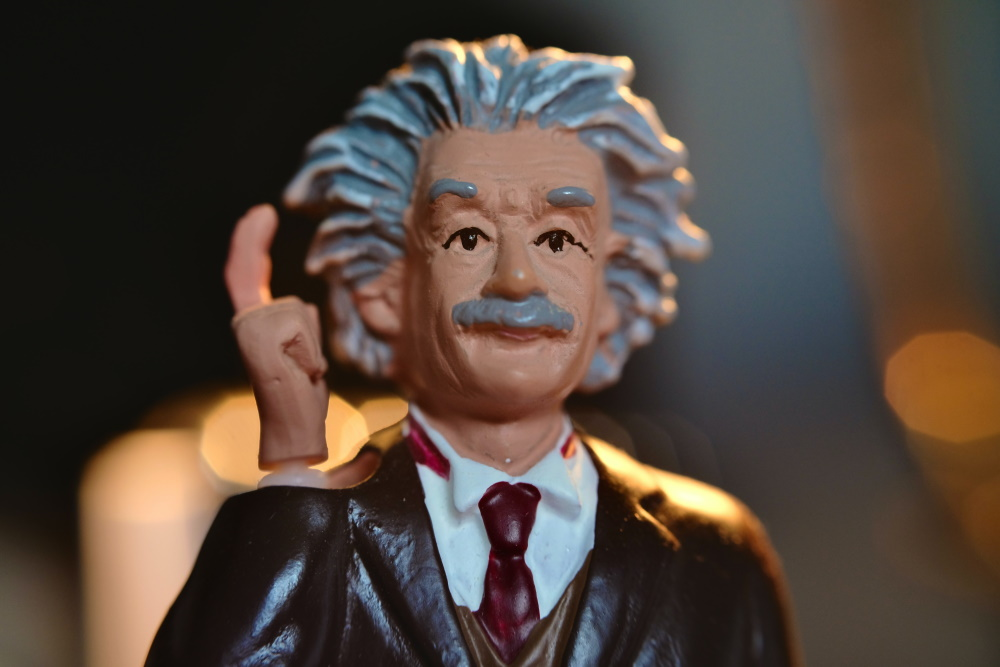The Full Story

Hi! I’m Will and I created a passive 5 figure passive income, within 5 years, through SEO and an effective blogging strategy. I share my incites exclusively on Ask Will Online.
dark

# Focus Length Of a Convex Lens: 1/V = 1/U +1/F

The focal length for convex lenses never changes with it being on the power of the lens. A convex is lens is thicker the further to the centre of the lens. Due to light travelling slower through glass, it forces the light waves to bend on the top and bottom of the lens producing a focus point in the image distance. We will also find out how to work out the U (object distance), V (image distance) and some important equations that students studying Physics AS will need to know. All will be explained better below.

Here is what happens to light when it enters a convex lens from infinite distance. It is at infinite distance or a distance where the light waves will be parallel to each other when entering the lens. This is where we can work out the focal length of a lens, only once the light going into the lens is of an infinite distance:

Here is what happens to light when it enters a convex from a closer distance. The light waves entering the lens are not parallel to each other causing the focus point to increase. The focal point never changes for a lens as shown below:
To work out the power of the lens or the curvature the lens adds to the light wave uses the equation:

Power = 1 / Focal Length in metres with Power being measured in dioptre (D)

Therefore, using symbols, the equation is P = 1 / F making P and F reciprocals of each other.
This means The more power you have in a lens, the more curvature that is added. As well as that, the higher the focal length, the lower the power (dioptre).

Understanding the object and image distances of a convex lens is also very important. The equation used to work out the image distance, object distance or focal length is:

1 / V = 1 / U + 1 / F (curvature after = curvature before + curvature added by lens)

• V is the distance from the lens to the image. It is measured in metres. It is always a positive number.
• U is the distance from object to the lens. It is also measured in metres. It is always a negative number.
• f is the focal length and is measured in dioptres.
There is all the theory to the lens and focal length. So, let’s test ourselves with a simple question:
If an object is 20cm from a lens and the image is 10cm from the lens, what is F? What is the power of the lens?
• First step, we should remind ourselves what equation we are going to use. We will use the 1/V = 1/U + 1/F.
• Next step, we will place the values we know into the equation remembering to convert the centimetres to metres. Therefore, the equation will not look like 1/0.1 = 1/-0.2 + 1/F.
• We need to rearrange the equation to make ‘1/F‘ the subject. To do this, we will minus the ‘1/-0.2‘ on both sides. This will make the equation now look like this 1/0.1 + 1/0.2 = 1/F (the 0.2 is positive now as we had to minus it when it was negative to get it on the correct side. Double negative = positive).
• Work out what U and V are so it looks like 10 + 5 = 1/F.
• We now have a reciprocal. 15 = 1/F or F = 1/15.
• F = 0.06m
• To work out the power of the lens, we will have to use Power = 1/F
• P = 1/0.06
• Power – 15 D

If you are revising Physics Topics, please have a look at other articles I have done on Physics you may be interested in.

1.Anonymous March 26, 2013
2.Will Green March 26, 2013

## Related Posts## Calculating the Radius of a Geostationary Orbit

To calculate the radius of a geostationary orbit, the centripetal force must equal the gravitational force on the satellite or mass.## Investigating Human Power – Physics IAA

Yes, another boring science IAA test. This time it's physics investigating human power by walking up stairs.

## Bits, Bytes and Binary Codes

All images and every digital sounds are made from ‘0’s and ‘1’s. Each one of these digits is…